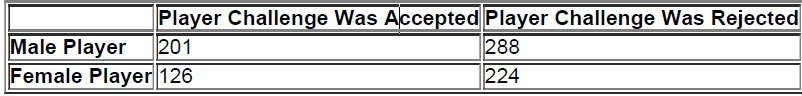×
Get Full Access to Elementary Statistics - 12 Edition - Chapter 4.8 - Problem 9cqq
Get Full Access to Elementary Statistics - 12 Edition - Chapter 4.8 - Problem 9cqq

×

# Use the following results from the 839player | Ch 4.8 - 9CQQISBN: 9780321836960 18

## Solution for problem 9CQQ Chapter 4.8

Elementary Statistics | 12th Edition

• Textbook Solutions
• 2901 Step-by-step solutions solved by professors and subject experts
• Get 24/7 help from StudySoup virtual teaching assistantsElementary Statistics | 12th Edition

4 5 1 380 Reviews
17
4
Problem 9CQQ

In Exercises 6–10, use the following results from the 839 player challenges to referee calls in the first U.S. Open tennis tournament to use the Hawk-Eye electronic instant replay system.Find the probability of randomly selecting 2 different challenges and finding that they were both accepted challenges made by female players.

Step-by-Step Solution:
Step 1 of 3

Solution  9CQQ

= 0.0224

Step 2 of 3

Step 3 of 3

##### ISBN: 9780321836960

Elementary Statistics was written by and is associated to the ISBN: 9780321836960. This full solution covers the following key subjects: challenges, use, open, accepted, cqq. This expansive textbook survival guide covers 121 chapters, and 3629 solutions. The full step-by-step solution to problem: 9CQQ from chapter: 4.8 was answered by , our top Statistics solution expert on 03/15/17, 10:30PM. The answer to “?In Exercises 6–10, use the following results from the 839 player challenges to referee calls in the first U.S. Open tennis tournament to use the Hawk-Eye electronic instant replay system. Find the probability of randomly selecting 2 different challenges and finding that they were both accepted challenges made by female players.” is broken down into a number of easy to follow steps, and 51 words. This textbook survival guide was created for the textbook: Elementary Statistics, edition: 12. Since the solution to 9CQQ from 4.8 chapter was answered, more than 522 students have viewed the full step-by-step answer.

## Discover and learn what students are asking

Calculus: Early Transcendental Functions : Graphs and Models
?In Exercises 5-14, sketch the graph of the equation by point plotting. $$y=(x-3)^{2}$$

Calculus: Early Transcendental Functions : Polar Equations of Conics and Keplers Laws
?Consider the polar equation $$r=\frac{4}{1-0.4 \cos \theta}$$ (a) Identify the conic without graphing the equation. (b) Wit

Statistics: Informed Decisions Using Data : The Design of Experiments
?What is replication in an experiment?

Unlock Textbook Solution

Enter your email below to unlock your verified solution to:

Use the following results from the 839player | Ch 4.8 - 9CQQ